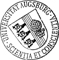## Optimal Control of Inductive Heating of Ferromagnetic Materials

• Inductive heating is a technological process where a steel workpiece is surrounded by an electromagnetic coil to which currents at various frequencies and time-varying amplitudes are applied. The amplitudes are considered as the controls and the objective is to heat the workpiece up to a desired temperature profile at the final time of the heating process. The workpiece is then quenched which due to a phase transition in the crystallographic structure of the steel leads to a hardening of the surface of the workpiece. For the inductive heating process, the state equations represent a coupled system of nonlinear partial differential equations consisting of the eddy currents equations in the coil, the workpiece, and the surrounding air, and a heat equation in the workpiece. The nonlinearity stems from the temperature dependent nonlinear material laws for steel both with regard to its electromagnetic and thermal behavior. Following the principle 'Discretize first, then optimize', weInductive heating is a technological process where a steel workpiece is surrounded by an electromagnetic coil to which currents at various frequencies and time-varying amplitudes are applied. The amplitudes are considered as the controls and the objective is to heat the workpiece up to a desired temperature profile at the final time of the heating process. The workpiece is then quenched which due to a phase transition in the crystallographic structure of the steel leads to a hardening of the surface of the workpiece. For the inductive heating process, the state equations represent a coupled system of nonlinear partial differential equations consisting of the eddy currents equations in the coil, the workpiece, and the surrounding air, and a heat equation in the workpiece. The nonlinearity stems from the temperature dependent nonlinear material laws for steel both with regard to its electromagnetic and thermal behavior. Following the principle 'Discretize first, then optimize', we consider a semi-discretization in time by the implicit Euler scheme which leads to a discrete-time optimal control problem. We prove the existence of a minimizer for the discrete-time optimal control problem and derive the first order necessary optimality conditions.Author: Oleg Boyarkin, Ronald H. W. HoppeGND urn:nbn:de:bvb:384-opus4-23389 https://opus.bibliothek.uni-augsburg.de/opus4/2338 Preprints des Instituts für Mathematik der Universität Augsburg (2013-10) Preprint English Universität Augsburg 2013/05/06 optimal control; inductive heating; eddy currents equations; heat equation Induktive Erwärmung; Wärmeleitungsgleichung; Wirbelstrom; Mathematische Modellierung; Optimale Kontrolle; Implizites Euler-Verfahren Mathematisch-Naturwissenschaftlich-Technische Fakultät Mathematisch-Naturwissenschaftlich-Technische Fakultät / Institut für Mathematik Mathematisch-Naturwissenschaftlich-Technische Fakultät / Institut für Mathematik / Lehrstuhl für Numerische Mathematik 5 Naturwissenschaften und Mathematik / 51 Mathematik / 510 MathematikDeutsches Urheberrecht mit Print on Demand